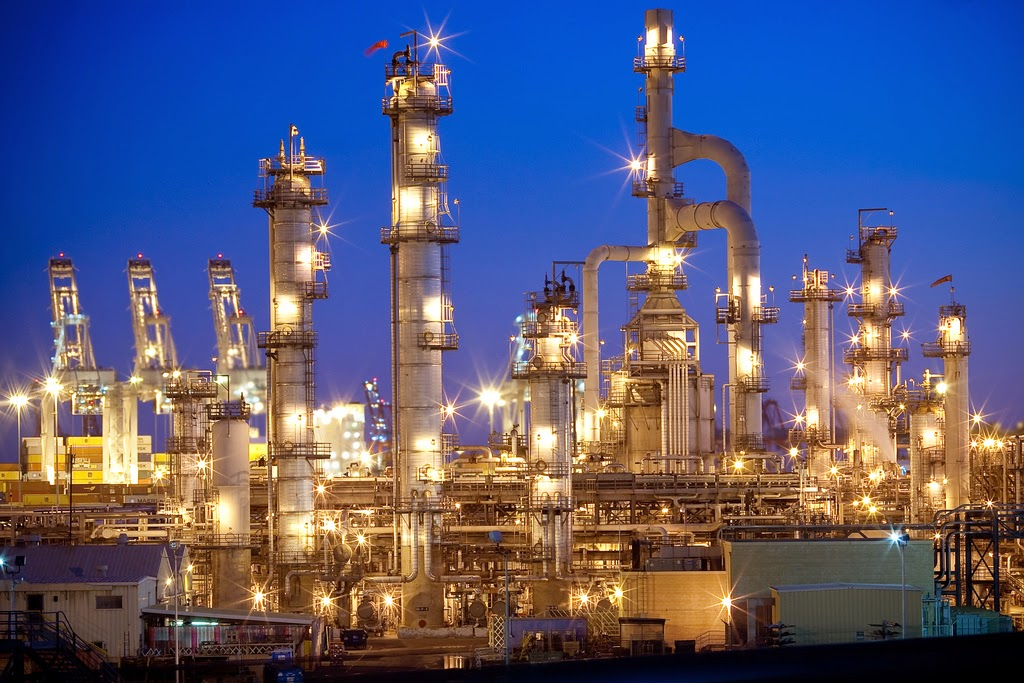## Top Ad unit 728 × 90

Oil and gas refinery facility - There are so many question came from IndustriMigas follower about how to estimating diameter size of distilation tower at refinery production facilities. Estimating diameter size of distillation tower in oil and gas refinery facility is the most important step to design a distillation tower. Detail steps of estimating diameters size of distillation coloumn are :Illustration Distillation Tower, doc. arb.ca.gov

## Calculate Liquid – Vapor Flow Parameter

To calculate liquid – vapour flow parameter (FLV) using this formula:

Where :
WL    = mass rate liquid per unit area, lb/ hr
Wv    = mass rate vapor per unit area, lb / hr
ρv     = density vapor ,lb/ft3
ρL     = density liquid, lb/ft3

## Calculate Corrected CSB

To calculate Corrected CSB using this formula:

Where :

σ      = actual interfacial tension (mN/m2)

## Calculate Vapor Velocity (UN)

To calculate vapor velocity using this formula:

## Calculate Net Area

To calculate net area of distillation tower using this formula :

Where :

AN     = Net area for vapor flow

CFS   = volumetric rate of vapor, ft3/sec

## Calculate Total Tower Area (AT)

To calculate total tower area of distillation tower using this formula :

Where :

## Calculate Diameter Tower (DT)

After calculate all of parameter above we can calculate Diameter of Distilation Tower using this formula :

Maximum clear liquid velocities in down comer*.

 Foaming Tendency Example Clear Liquid Velocity, ft/sec Spacing 18 in 24 in 30 in Low Low press. (<100 psia).Light HC. Stabilizer. air-water simulator 0.4-0.5 0.5-0.6 0.6-0.7 Medium Oil system. crude oil distillation. mid press. (100-300 psia) HC 0.3-0.4 0.4-0.5 0.6-0.7 High Amine. glycerine. glycols. high press. (>300 psia). light HC 0.2-0.25 0.2-0.25 0.2-0.3

Note : * Source “Distillation Operation. Henry Z. Kister”
How to Estimating Diameter Size of Distilation TowerReviewed by Djoernalist on 9:45 AM Rating: 5

Leave your comment on this text box